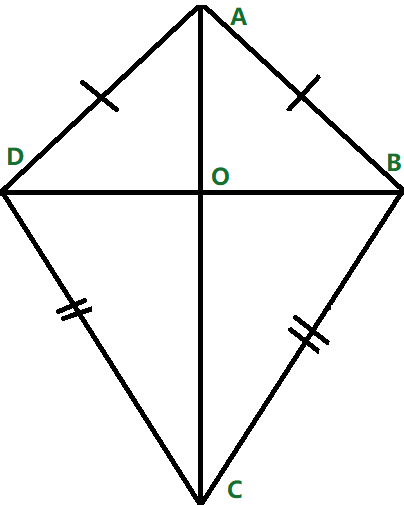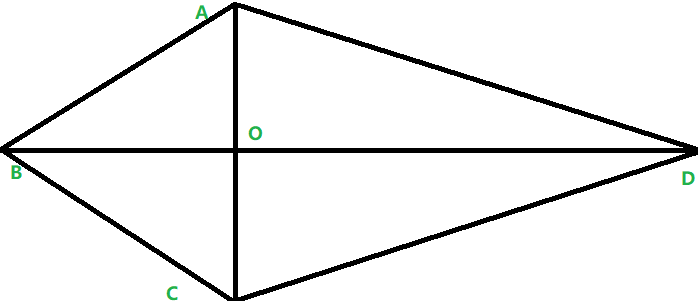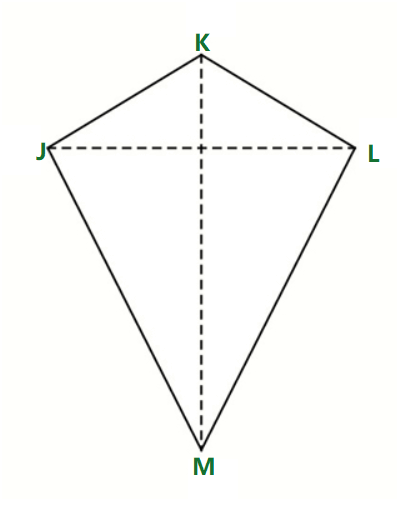Related Articles

A Kite is a quadrilateral in which four sides can be grouped into two pairs of equal-length sides that are adjacent to each other and the diagonals intersect each other at right angles. The quadrilateral is a 4-sided polygon. The figure shown below represents a kite:### Properties of Kite

1. Kite has 2 diagonals that intersect each other at right angles.
2. A kite is symmetrical about its main diagonal.
3. Angles opposite to the main diagonal are equal.
4. The kite can be viewed as a pair of congruent triangles with a common base.
5. The shorter diagonal divides the kite into 2 isosceles triangles.
6. The area of the kite is 1/2 × d1 × d2

### To Prove: The diagonals of kite intersect at right angles (are perpendicular).Proof:

In the figure given above, in ∆ABD and ∆BCD

AB = BC (property of kite)

AD = CD (property of kite)

BD = BD (common side)

Hence ∆ABD ≅ ∆BCD (SSS congruency)

AB = BC (property of kite)

Hence  ∆ABC is an isosceles triangle.

AD = CD (property of kite)

Hence ∆ADC is an isosceles triangle.

∠BAO = ∠BCO

BO = BO (common side)

Thus, ∆ABO ≅ ∆BCO (SAS rule of congruency)

Now we know ∠AOB = ∠BOC

Also, ∠AOB + ∠BOC = 180° (Linear pair)

Hence, ∠AOB = ∠BOC = 90°

Hence diagonals of kite intersect at right angles.

## Solved Examples on Kite

Example 1: Find the area of a kite whose diagonals are 40 cm and 35 cm.

Solution:

The area of the kite with diagonals as d1 and d2 is given as 1/2 × d1 × d2.

Area = 1/2 × 40 × 35

Area = 700 cm2

Hence the area is 700 cm2

Example 2: Find the unknown angles of the given kite.

Given that:

• ∠JKL = 100
• ∠KJL = 40Solution:

As we know that the main diagonal bisects the kite into two halves.

Hence the angles ∠KJL = ∠KLM

Hence ∠KLM = 100

Also since the sum of all angles of the quadrilateral is 360.

Hence ∠JML = 120

Example 3: The Area of a kite-shaped field is 450 cm² and the length of one of its diagonal is 50 cm. A man wants to cross the field through the other diagonal. Find the distance the man has to travel.

Solution:

Given,

Area of a kite = 450 cm²

Length of one diagonal = 50 cm

We know that the Area of Kite = 1/2 × d1 × d2

450 = 1/2 × 50 × d2

d2 = 18 cm

Hence the other man has to travel a distance of 18 cm.

Whether you're preparing for your first job interview or aiming to upskill in this ever-evolving tech landscape, GeeksforGeeks Courses are your key to success. We provide top-quality content at affordable prices, all geared towards accelerating your growth in a time-bound manner. Join the millions we've already empowered, and we're here to do the same for you. Don't miss out - check it out now!

Previous
Next